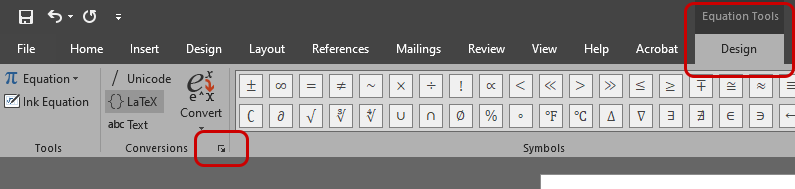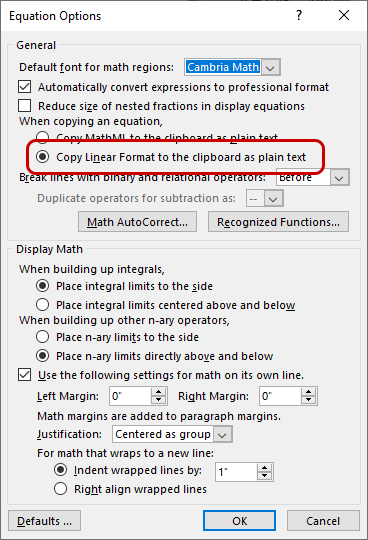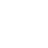# Accessible Mathematic Notation on Webpages

LaTeX notation in website content can be re-formatted automatically with the MathJax JavaScript library. This can create visually formatted math expressions that are compatible with most browsers and screen readers.

## Copy LaTeX notation from MS Word Equations

Note: requires a current desktop version of MS Word/Office 365. See MS documentation about writing equations and formulas.

1. While in the Equation Tools - Design toolbar, click the small boxed arrow icon in the Conversions group:2. For the setting labeled "When copying an equation", select "Copy Linear Format to the clipboard as plain text":3. Now when you copy an equation, the clipboard will contain the plaintext Linear/LaTeX notation

For Cascade pages, include the MathJax.js asset from the global site.

For other web systems, load the MathJax script from a CDN.

## Mark LaTeX notation for MathJax formatting:

Before MathJax can work to transform LaTeX, you must mark each piece of math notation.

### Block Layout: \$... \$

Wrap in backslash and square brackets for block layout (centered, separate section):

$$x=\frac{-b\pm\sqrt{b^2-4ac}}{2a}$$

### Inline Layout: \$$... \$$

Wrap in backslash and parenthesis for inline layout (flows within sentence):

When $$a \ne 0$$, there are two solutions to $$ax^2 + bx + c = 0$$